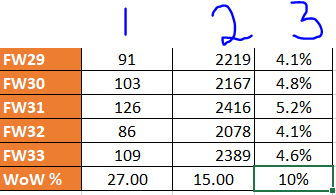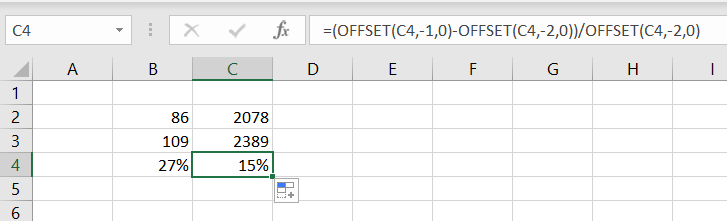# Calculate value of Dynamically changing cell

Hi,

I have an excel file that dynamically updates. (for example, when there is data for week 34 (FW34), it dynamically adds it above WoW%

each time this happens, I would like to perform a calculation and write the result on WoW%'s row

for example
(109/86)/86 = 27 which is (FW33(Column1)/FW32(Column1))/FW32(Column1)

And this applies to column 2 too

I would appreciate any help

Thank youThis should do the work:

``````=(OFFSET(B4,-1,0)-OFFSET(B4,-2,0))/OFFSET(B4,-2,0)
``````

You just have to replace “B4” with your cell reference.

Same for C column:Best,
Charbel